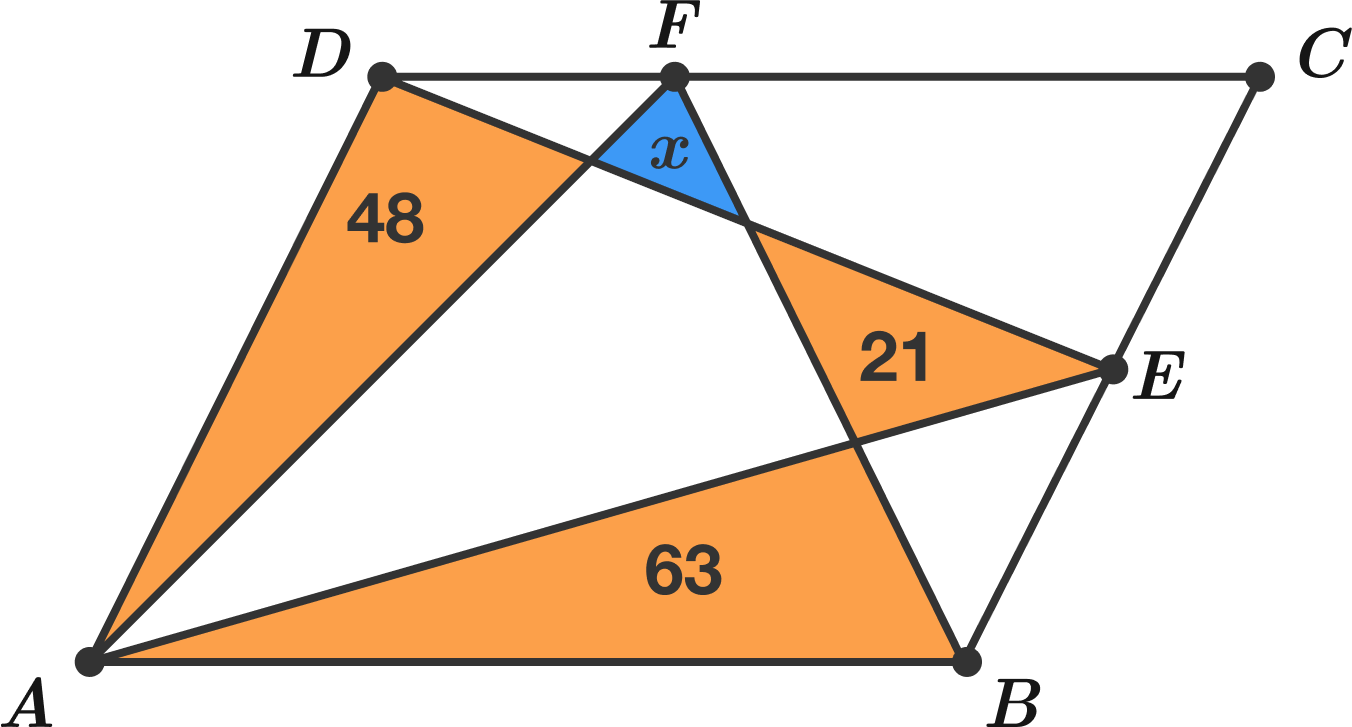# What is the area of the blue triangle?

Geometry Level 2

The line segments shown divide parallelogram $ABCD$ into 8 regions, and the areas of the orange triangles are 48, 21, and 63.

What is the area of the blue triangle?×